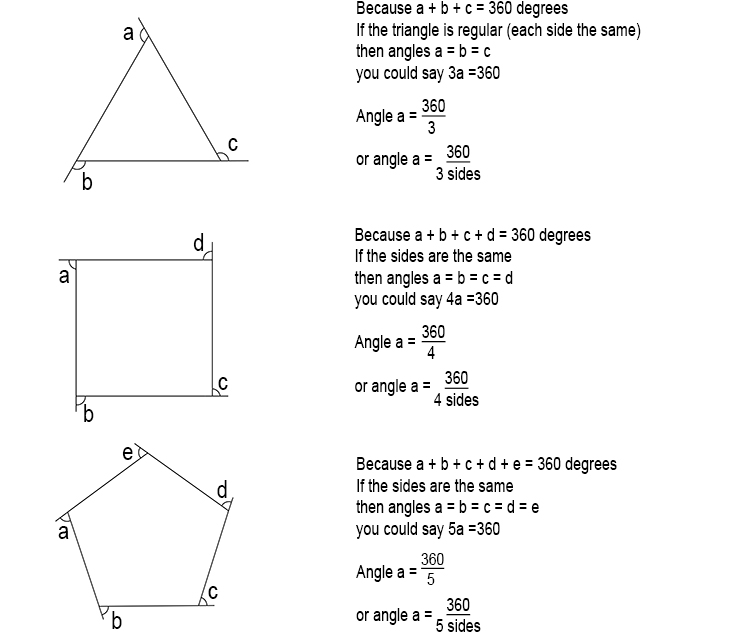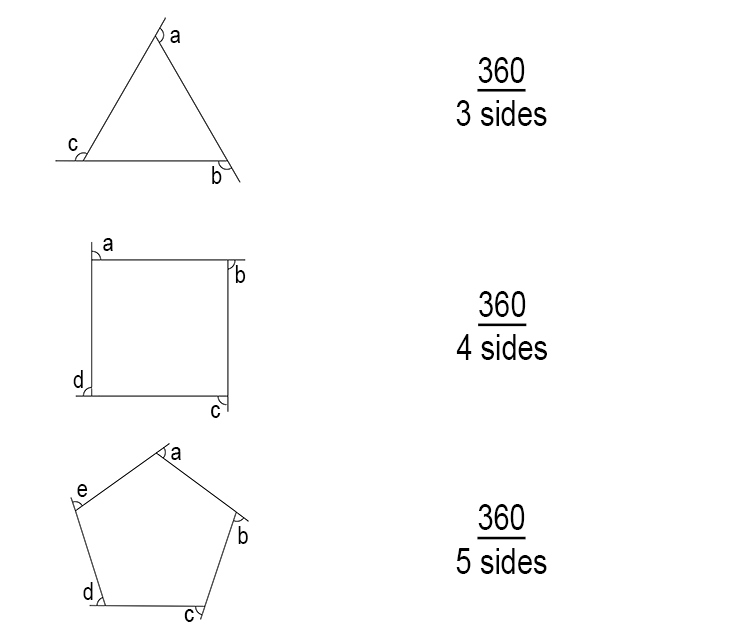# External Angles of a Regular Polygon

To remember the formula for the external angle of a regular (each side the same) polygon, you should always work out the first three polygons, i.e triangle, square and pentagon, and remember that the total sum of all external angles of a polygon = 360°.Therefore, the size of one external angle of regular (each side the same) polygon is

=360/(Number  of  sides)

which is often shown as

=360/n

where n is the number of sides.

Note that this works both ways:And again, the external angle of these regular polygons

=360/(Number  of  sides)